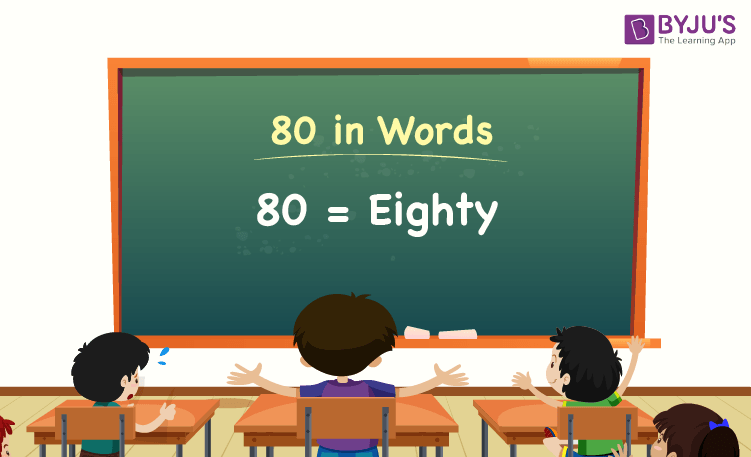# 80 in Words

80 in words is Eighty. For example, if you spent Rs. 80 this month, you can write, “I have spent Rs. Eighty this month”. The place value chart helps to write the number name of 80.

 80 in Words: Eighty

In this article, we will discuss how to write the number 80 in words, and look at many solved examples in detail.

## How to Write 80 in Words?The number 80 in words is written using the place value of a number. Thus, to write 80 in words, find the place value of each digit of 80.

For a number 80,

The digit in unit/one’s place = 0

The digit in ten’s place = 8

Since the number 80 is a two-digit number, there are no hundreds and thousands.

Thus, the place value chart for 80 is:

 Tens Ones 8 0

Therefore, the number 80 in words is Eighty.

### Examples

Example 1:

Express the value of 50 + 30 in words.

Solution:

Given expression: 50 + 30

⇒ 50 + 30 = 80

So, the value of 50 + 30 is 80.

Hence, 80 in words is eighty.

Example 2:

Find the value of one hundred plus eighty, and express it in words.

Solution:

One hundred = 100

Eighty = 80

One hundred plus eighty = 100 + 80 = 180

Hence, the value of one hundred plus eighty is one hundred eighty in words.

## Frequently Asked Questions on 80 in Words

Q1

### Write 80 in words.

80 in words is eighty.

Q2

### Find the value of 100 – 20, and express the answer in words.

Simplifying 100 – 20, we get 80. Hence, 80 in words is eighty.

Q3

### How to write eighty in numbers?

Eighty in numbers is 80.

Q4

### Is 80 an even number?

Yes, 80 is an even number.

Q5

### Is 80 a perfect square number?

No, 80 is not a perfect square number.